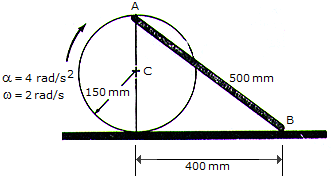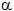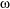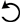# Engineering Mechanics - Planar Kinematics of a Rigid Body (PKRB) - Discussion

### Discussion :: Planar Kinematics of a Rigid Body (PKRB) - General Questions (Q.No.16)

16.The disk rolls without slipping such that it has an angular acceleration of= 4 rad/s2 and angular velocity of= 2 rad/s at the instant shown. Determine the accelerations of points A and B on the link and the link's angular acceleration at this instant. Assume point A lies on the periphery of the disk, 150 mm from C.

 [A]. aA = (1.200i - 0.600j) m/s2, aB = 1.650i m/s2, "AB = 1.500 rad/s2[B]. aA = (1.200i - 0.600j) m/s2, aB = 1.650i m/s2, "AB = 1.500 rad/s2[C]. aA = (1.200i - 2.40j) m/s2, aB = 3.00i m/s2, "AB = 6.00 rad/s2[D]. aA = (1.200i - 1.200j) m/s2, aB = 2.10i m/s2, "AB = 3.00 rad/s2Explanation:

No answer description available for this question.

 Carlos said: (Dec 7, 2014) How do we get the angular velocity of the link AB?

 Niran said: (Feb 14, 2017) Please give the description of the answer.